﻿ TSI阵风作用下箱梁断面的气动特性分析 Aerodynamic Characteristic Analysis of Box Girder Section in TSI Gusty Wind

Modeling and Simulation
Vol. 09  No. 01 ( 2020 ), Article ID: 33495 , 10 pages
10.12677/MOS.2020.91003

Aerodynamic Characteristic Analysis of Box Girder Section in TSI Gusty Wind

Fei Huang, Lin Huang*, Junji Zhou

School of Civil Engineering, Architecture and Environment, Xihua University, Chengdu Sichuan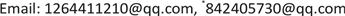Received: Nov. 27th, 2019; accepted: Dec. 10th, 2019; published: Dec. 17th, 2019ABSTRACT

The aerodynamic forces on box girder section and flow field are simulated by CFD (computational fluid dynamics) method in TSI (Technical Specifications for Interoperability) gusty wind at the angles of attack from −5˚ to +5˚. Reynolds-averaged Navier-Stokes (RANS) equations for 2D incompressible unsteady flow are integrated with RNG (Renormalized Group) k-ε turbulence model in this research. The time histories of the aerodynamic forces on the box girder section in TSI gusty wind slowly fluctuate around the reference values based on the quasi-steady assumption, but the oscillation amplitudes at high angles of attack are relatively large. The sudden change of drag coefficient, the change of lift coefficient lagging the wind speed of the TSI gust, and the favorable and adverse pressure gradients are observed and analyzed.

Keywords:TSI Gusty Wind, Box Girder Section, Aerodynamic Characteristic, Numerical SimulationTSI阵风作用下箱梁断面的气动特性分析1. 引言

2. 物理模型

2.1. 阵风模型

$V\left(t\right)=\beta \left(t\right)U$ (1)

$\beta \left(t\right)=\left\{\begin{array}{l}A+\left(A-1\right)\text{sin}\left(\pi t+0.5\pi \right)\text{\hspace{0.17em}}\text{\hspace{0.17em}}\text{\hspace{0.17em}}\text{\hspace{0.17em}}\text{\hspace{0.17em}}\text{\hspace{0.17em}}\text{\hspace{0.17em}}\text{\hspace{0.17em}}\text{\hspace{0.17em}}\text{\hspace{0.17em}}\text{\hspace{0.17em}}\text{\hspace{0.17em}}\text{\hspace{0.17em}}\text{\hspace{0.17em}}\text{\hspace{0.17em}}\text{\hspace{0.17em}}\text{\hspace{0.17em}}1\le t\le 1.5\\ A+\left(A-1\right)\text{sin}\left(\pi t-0.5\pi \right)\text{\hspace{0.17em}}\text{\hspace{0.17em}}\text{\hspace{0.17em}}\text{\hspace{0.17em}}\text{\hspace{0.17em}}\text{\hspace{0.17em}}\text{\hspace{0.17em}}\text{\hspace{0.17em}}\text{\hspace{0.17em}}\text{\hspace{0.17em}}\text{\hspace{0.17em}}\text{\hspace{0.17em}}\text{\hspace{0.17em}}\text{\hspace{0.17em}}\text{\hspace{0.17em}}\text{\hspace{0.17em}}\text{\hspace{0.17em}}1.5\le t\le 2\\ 2-A-\left(A-1\right)\text{sin}\left(\pi t-1.5\pi \right)\text{\hspace{0.17em}}\text{\hspace{0.17em}}\text{\hspace{0.17em}}\text{\hspace{0.17em}}\text{\hspace{0.17em}}\text{\hspace{0.17em}}\text{\hspace{0.17em}}\text{\hspace{0.17em}}\text{\hspace{0.17em}}\text{\hspace{0.17em}}\text{\hspace{0.17em}}\text{ }\text{ }\text{\hspace{0.17em}}3\le t\le 3.5\\ 2-A+\left(A-1\right)\text{sin}\left(\pi t+2.5\pi \right)\text{\hspace{0.17em}}\text{\hspace{0.17em}}\text{\hspace{0.17em}}\text{\hspace{0.17em}}\text{\hspace{0.17em}}\text{\hspace{0.17em}}\text{\hspace{0.17em}}\text{\hspace{0.17em}}\text{\hspace{0.17em}}\text{\hspace{0.17em}}\text{\hspace{0.17em}}\text{\hspace{0.17em}}3.5\le t\le 4\\ 1.0\text{\hspace{0.17em}}\text{\hspace{0.17em}}\text{\hspace{0.17em}}\text{\hspace{0.17em}}\text{\hspace{0.17em}}\text{\hspace{0.17em}}\text{\hspace{0.17em}}\text{\hspace{0.17em}}\text{\hspace{0.17em}}\text{\hspace{0.17em}}\text{\hspace{0.17em}}\text{\hspace{0.17em}}\text{\hspace{0.17em}}\text{\hspace{0.17em}}\text{\hspace{0.17em}}\text{\hspace{0.17em}}\text{\hspace{0.17em}}\text{\hspace{0.17em}}\text{\hspace{0.17em}}\text{\hspace{0.17em}}\text{\hspace{0.17em}}\text{\hspace{0.17em}}\text{\hspace{0.17em}}\text{\hspace{0.17em}}\text{\hspace{0.17em}}\text{\hspace{0.17em}}\text{\hspace{0.17em}}\text{\hspace{0.17em}}\text{\hspace{0.17em}}\text{\hspace{0.17em}}\text{\hspace{0.17em}}\text{\hspace{0.17em}}\text{\hspace{0.17em}}\text{\hspace{0.17em}}\text{\hspace{0.17em}}\text{\hspace{0.17em}}\text{\hspace{0.17em}}\text{\hspace{0.17em}}\text{\hspace{0.17em}}\text{\hspace{0.17em}}\text{\hspace{0.17em}}\text{\hspace{0.17em}}\text{\hspace{0.17em}}\text{\hspace{0.17em}}\text{\hspace{0.17em}}\text{\hspace{0.17em}}t\text{ }取其它值\end{array}$ (2)

2.2. 箱梁模型及计算域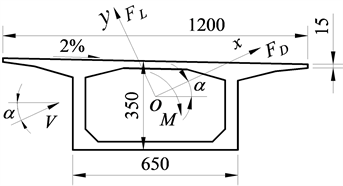Figure 1. The section of box girder (unit: cm)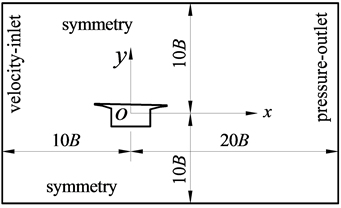Figure 2. Numerical simulation domain

2.3. 气动力

${C}_{D}\left(\alpha \right)={F}_{D}\left(\alpha \right)/\left(0.5\rho {U}^{2}BL\right)$ (3a)

${C}_{L}\left(\alpha \right)={F}_{L}\left(\alpha \right)/\left(0.5\rho {U}^{2}BL\right)$ (3b)

${C}_{M}\left(\alpha \right)=M\left(\alpha \right)/\left(0.5\rho {U}^{2}{B}^{2}L\right)$ (3c)

${C}_{D,G}\left(\alpha ,t\right)={F}_{D,G}\left(\alpha ,t\right)/\left(0.5\rho {U}^{2}BL\right)$ (4a)

${C}_{L,G}\left(\alpha ,t\right)={F}_{L,G}\left(\alpha ,t\right)/\left(0.5\rho {U}^{2}BL\right)$ (4b)

${C}_{M,G}\left(\alpha ,t\right)={M}_{G}\left(\alpha ,t\right)/\left(0.5\rho {U}^{2}{B}^{2}L\right)$ (4c)

${C}_{D,G0}\left(\alpha ,t\right)={\beta }^{2}\left(t\right){C}_{D}\left(\alpha \right)$ (5a)

${C}_{L,G0}\left(\alpha ,t\right)={\beta }^{2}\left(t\right){C}_{L}\left(\alpha \right)$ (5b)

${C}_{M,G0}\left(\alpha ,t\right)={\beta }^{2}\left(t\right){C}_{M}\left(\alpha \right)$ (5c)

3. 数学模型

3.1. 控制方程

$\frac{\partial u}{\partial x}+\frac{\partial v}{\partial y}=0$ (6a)

$\rho \left(\frac{\partial u}{\partial t}+u\frac{\partial u}{\partial x}+v\frac{\partial u}{\partial y}\right)=-\frac{\partial \stackrel{˜}{p}}{\partial x}+\frac{\partial }{\partial x}\left({\mu }_{\text{ef}}\frac{\partial u}{\partial x}\right)+\frac{\partial }{\partial y}\left({\mu }_{\text{ef}}\frac{\partial u}{\partial y}\right)$ (6b)

$\rho \left(\frac{\partial v}{\partial t}+u\frac{\partial v}{\partial x}+v\frac{\partial v}{\partial y}\right)=-\frac{\partial \stackrel{˜}{p}}{\partial x}+\frac{\partial }{\partial x}\left({\mu }_{\text{ef}}\frac{\partial v}{\partial x}\right)+\frac{\partial }{\partial y}\left({\mu }_{\text{ef}}\frac{\partial v}{\partial y}\right)$ (6c)

k和 ${\mu }_{t}$ 由RNG k-ε湍流模型的运输方程  确定：

$\rho \left(\frac{\partial k}{\partial t}+u\frac{\partial k}{\partial x}+v\frac{\partial k}{\partial y}\right)=\frac{\partial }{\partial x}\left({\alpha }_{k}{\mu }_{\text{ef}}\frac{\partial k}{\partial x}\right)+\frac{\partial }{\partial y}\left({\alpha }_{k}{\mu }_{\text{ef}}\frac{\partial k}{\partial y}\right)+{S}_{k}$ (7a)

$\rho \left(\frac{\partial \epsilon }{\partial t}+u\frac{\partial \epsilon }{\partial x}+v\frac{\partial \epsilon }{\partial y}\right)=\frac{\partial }{\partial x}\left({\alpha }_{\epsilon }{\mu }_{\text{ef}}\frac{\partial \epsilon }{\partial x}\right)+\frac{\partial }{\partial y}\left({\alpha }_{\epsilon }{\mu }_{\text{ef}}\frac{\partial \epsilon }{\partial y}\right)+{S}_{\epsilon }-{R}_{\epsilon }$ (7c)

${C}_{1\epsilon }=1.42$${C}_{2\epsilon }=1.68$${C}_{\upsilon }=100$${\alpha }_{0}=1.0$${\eta }_{0}=4.38$$\beta \approx 0.012$${C}_{\mu }=0.0845$

3.2. 计算网格及时间步长(a) Grid 1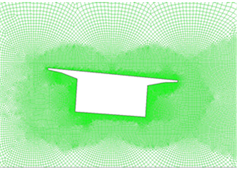(b) Grid 2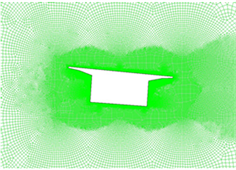(c) Grid 3

Figure 3. Local meshes of three gird schemesTable 1. Comparison of the CFD results and wind tunnel test data of static coefficients of aerodynamic forcesTable 2. Numerical results of static coefficients of aerodynamic forces at different iteration time steps

4. 箱梁断面的静力三分力系数验证Table 3. Comparison of CFD results and wind tunnel data of static coefficients of aerodynamic forces

5. TSI阵风作用下箱梁断面的气动力及流场特性

5.1. 箱梁断面的气动力特性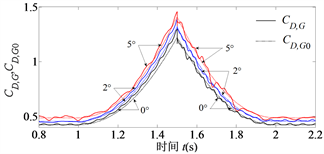(a)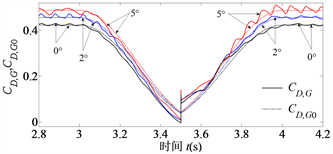(b)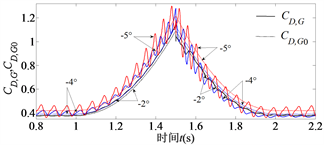(c)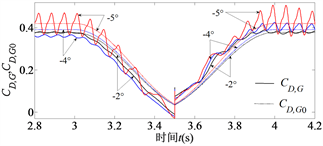(d)

(a)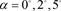and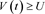(b)and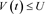(c)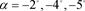and(d)andFigure 4. Time history of the coefficient of drag in TSI gusty wind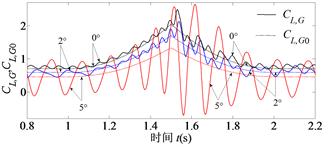(a)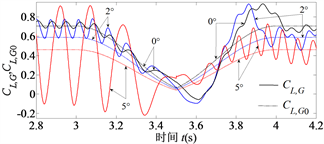(b)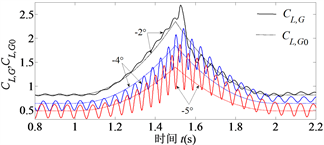(c)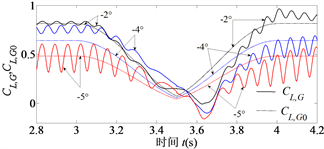(d)

(a)and(b)and(c)and(d)andFigure 5. Time history of the coefficient of lift in TSI gusty wind

5.2. 箱梁断面的绕流流场特性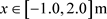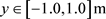)所示。箱梁断面的气动力计算仅考虑箱梁表面的静压的相对值，故流

(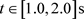)，箱梁表面的远场静压随计算时间增大而增大。当入口风速由10 m/s逐步增大到最大值17 m/s时(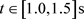)，CFD计算得到的入口静压由0 Pa逐渐增大到约245 Pa。当入口风速达到最大值

17 m/s后，再计算1个迭代时间步，入口静压急剧下跌到约−480 Pa；然后，入口风速逐步减小到10 m/s

0 Pa。此处的入口静压跳跃也是前述箱梁断面的阻力系数在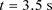跳跃的原因。(a)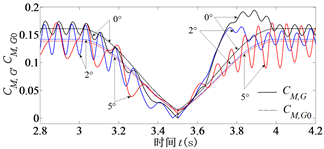(b)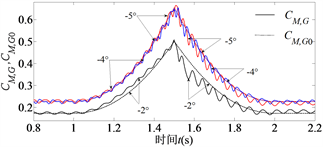(c)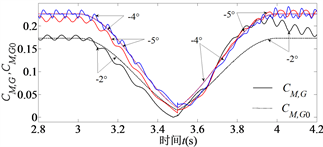(d)

(a)and(b)and(c)and(d)andFigure 6. Time history of the coefficient of moment in TSI gusty wind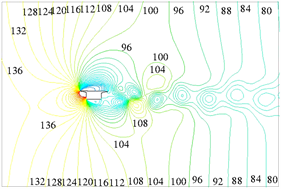(a)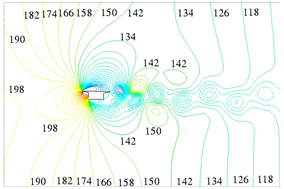(b)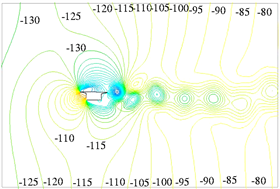(c)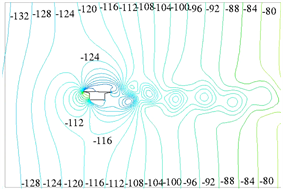(d)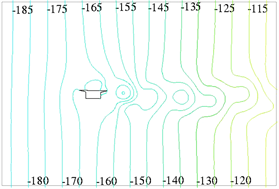(e)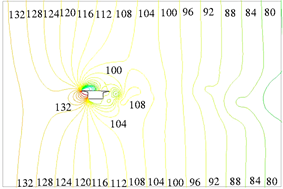(f)

(a)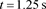(b)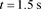(c)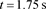(d)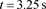(e)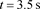(f)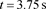Figure 7. Static pressure distribution at angle of attack of 0˚ (unit: Pa)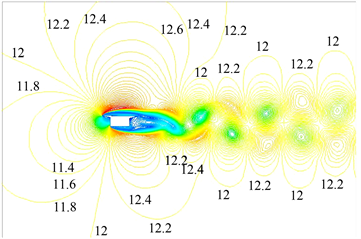(a)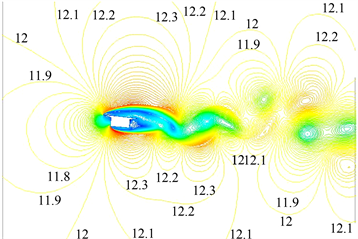(b)

(a)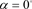(b)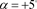Figure 8. Comparison of velocity distribution at angles of attack of 0˚ and +5˚ at(unit: m/s)

6. 结论

Aerodynamic Characteristic Analysis of Box Girder Section in TSI Gusty Wind[J]. 建模与仿真, 2020, 09(01): 19-28. https://doi.org/10.12677/MOS.2020.91003

1. 1. 同济大学. JTG/T 3360-01-2018公路桥梁抗风设计规范[S]. 北京: 人民交通出版社股份有限公司, 2018.

2. 2. 陈艾荣, 黄鹏, 项海帆. 桥梁阵风风速系数研究[J]. 同济大学学报, 1998, 26(3): 241-244.

3. 3. Xu, Y.L. and Ding Q.S. (2006) Interaction of Railway Vehicles with Track in Cross-Winds. Journal of Fluids and Structures, 22, 295-314. https://doi.org/10.1016/j.jfluidstructs.2005.11.003

4. 4. BSI Standards Limited (2018) BS EN 14067-6 Railway Applications-Aerodynamics, Part 6: Requirements and Test Procedures for Cross Wind Assessment.

5. 5. Proppe, C. and Wetzel, C. (2010) A Probabilisitic Approach for Assessing the Crosswind Stability of Ground Vehicles. Vehicle System Dynamics: International Journal of Vehicle Mechanics and Mobility, 48, 411-428. https://doi.org/10.1080/00423114.2010.482158

6. 6. 曹亚博, 凌亮, 邓永权, 等. 强阵风环境下高速列车运行安全性研究[J]. 机械工程学报, 2013, 49(18): 30-37.

7. 7. 吴超, 杜礼明. 瞬态风场下带风屏障的高架桥上高速列车气动特性[J]. 大连交通大学学报, 2017, 38(2): 21-26.

8. 8. Taylor, G.I. (1938) The Spectrum of Turbulence. Pro-ceedings of the Royal Society of London. Series A, Mathematical and Physical Sciences, 164, 476-490. https://www.jstor.org/stable/97077 https://doi.org/10.1098/rspa.1938.0032

9. 9. Carrarini, A. (2006) Relia-bility Based Analysis of the Crosswind Stability of Railway Vehicles. Ph.D. Thesis, Technical University of Berlin, Berlin.

10. 10. 刘钥, 陈政清, 张志田. 箱梁断面静风力系数的CFD数值模拟[J]. 振动与冲击, 2010, 29(1): 133-137.

11. 11. Yakhot, V. and Orszag, S.A. (1986) Renormalization Group Analysis of Turbulence I. Basic Theory. Journal of Scientific Computing, 1, 3-51. https://doi.org/10.1007/BF01061452

12. 12. 黄林. 计算流体力学在桥梁风工程中的应用研究[M]. 成都: 西南交通大学出版社, 2017.

13. 13. Huang, L., Liao, H.L., Wang, B., et al. (2009) Numerical Simulation for Aerodynamic Derivatives of Bridge Deck. Simulation Modelling Practice and Theory, 17, 719-729. https://doi.org/10.1016/j.simpat.2008.12.004

14. NOTES

*通讯作者。